by: Rudy C Tarumingkeng
Reference: Edelstein-Keshet, L (1988): Mathematical Models in Biology.
Random House, New York. p. 218-219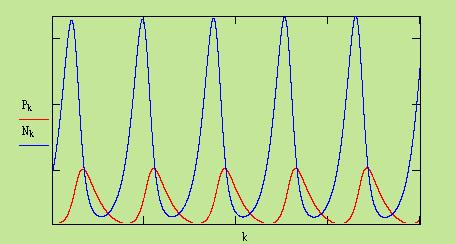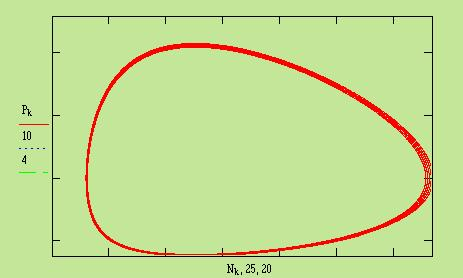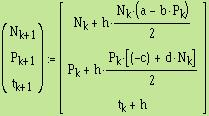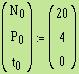The equations along with the time are converted into the following sumultaneous descrete equation: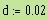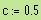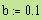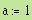Values given for a, b, c and d: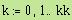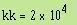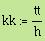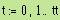h = step,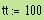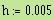This is a simulation of the Lotka-Volterra two species interaction (i.e. predator and prey) with simple assumptions as follow (Edelstein-Keshet, 1988):
1. Prey population grow in an unlimited way when there is no predators
2. For the prey to survive predator must be present
3. The rate of predation depends on the likelihood that a victim is encountered by a predator
4. The growth rate of predator is proportional to the rate of predation (food intake).

Lotka-Volterra model:

(N) dN/dt = aN - bNP (prey population)
(P) dP/dt = -cP + dPN (predator population)
a, b, c and d > 0

Using Euler method does not give exact results but it shows the cycle approximation.

a = growth rate of prey in the absence of predator
c = death rate of predator in the absence of prey ,
b = predator efficiency in causing prey population decline
bP = death rate of prey due to predation
d = prey efficiency in contributing to predator population growth
dN = growth rate of predator due to feeding on prey
FILE LV-EU: oleh Rudy C Tarumingkeng, PhD
JUNI 1996
INTERAKSI DUA SISTEM, MODEL LOTKA-VOLTERRA
SOLUSI DENGAN METODA EULER: DERET TAYLOR SAMPAI SUKU KEDUA